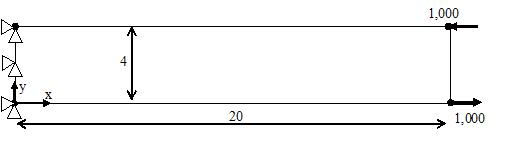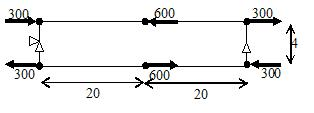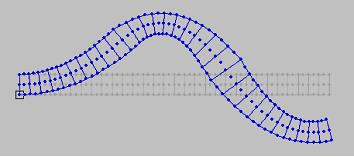Back to Assignments

## Assignments: Bending

Once you have downloaded the .pdf or .doc file and you are ready to do the assignment, you must start the program by clicking on 'Run Program.' Before you run the program, be sure you have gone through the CMU Mini-FEA Tutorial.

## Brief Description of Assignments:

4.1.1
(i) Conduct FEA for long slender bar with displacements fixed at one end (cantilevered), and equal and opposite forces at other end (moment); (ii) compare FEA normal stress at various points in one cross-section with bending theory; (iii) find deflection, slope (finite difference of displacement at neighboring nodes), and curvature (finite difference of slope at neighboring nodes); (iv) compare curvature with M/EI.Full Assignment 4.1.1(.pdf) Full Assignment 4.1.1 (.doc)

4.1.2
(i) Conduct FEA for long slender bar with equal couples at two ends, and balancing couple in center; (ii) compare FEA normal stress at various points in one cross-section with bending theory; (iii) find deflection, slope (finite difference of displacement at neighboring nodes), and curvature (finite difference of slope at neighboring nodes) and compare with M/EI.Full Assignment 4.1.2(.pdf) Full Assignment 4.1.2 (.doc)

4.1.3
(i) Student is given the deformed mesh, and partial information on loading: the bar is fixed at one end, has three applied couples (known magnitudes, unknown locations and senses), and has a specified maximum bending stress. By studying the deformed mesh, the student can determine the direction of the curvatures, and hence, the location and senses of the couples, and compare FEA normal stresses with predictions of beam theory.Full Assignment 4.1.3(.pdf) Full Assignment 4.1.3 (.doc)

Back to Assignments